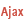HelpWLanguageWLanguage functionsStandard functionsNumeric values management functionsMatrix functionsMatReadPresentationExampleMatrix existenceManaging errorsSee alsoMatAdd (Example)MatAddMatCopy (Example)MatCopyMatCreate (Example)MatCreateMatDelete (Example)MatDeleteMatDeterminant (Example)MatDeterminantMatError (Example)MatErrorMatExist (Example)MatExistMatFill (Example)MatFillMatFloatAdd (Example)MatFloatAddMatFloatMultiply (Example)MatFloatMultiplyMatInvert (Example)MatInvertMatMultiply (Example)MatMultiplyMatNbColumn (Example)MatNbColumnMatNbLine (Example)MatNbLineMatRead (Example)MatReadMatReadColumn (Example)MatReadColumnMatReadLine (Example)MatReadLineMatStack (Example)MatStackMatTranspose (Example)MatTransposeMatWrite (Example)MatWriteMatrix management functionsThe matrices
 Matrix existenceManaging errors WINDEVWEBDEVWINDEV MobileOthersMatRead (Function) In french: MatLitNot available Reads the value of a matrix element.Reminder: A matrix element is identified by its row and by its column.Example // Read the element (row 3, column 5)ResRead = MatRead("MyMatrix", 3, 5) Syntax = MatRead( , , ) : RealValue of element read. : Character stringName of the matrix to be read, defined with MatCreate. : IntegerNumber of the row for the element to read. : IntegerNumber of the column for the element to read. Remarks Matrix existenceThe matrix existence is not checked. To check the matrix existence, use MatExist.Managing errorsCaution: MatRead returns no error code. To find out whether errors have been generated when reading an element, use MatError. To get more details on the error, use ErrorInfo with the errMessage constant.Business / UI classification: Neutral codeComponent: wd270mat.dll Minimum version required Version 9 This page is also available for…